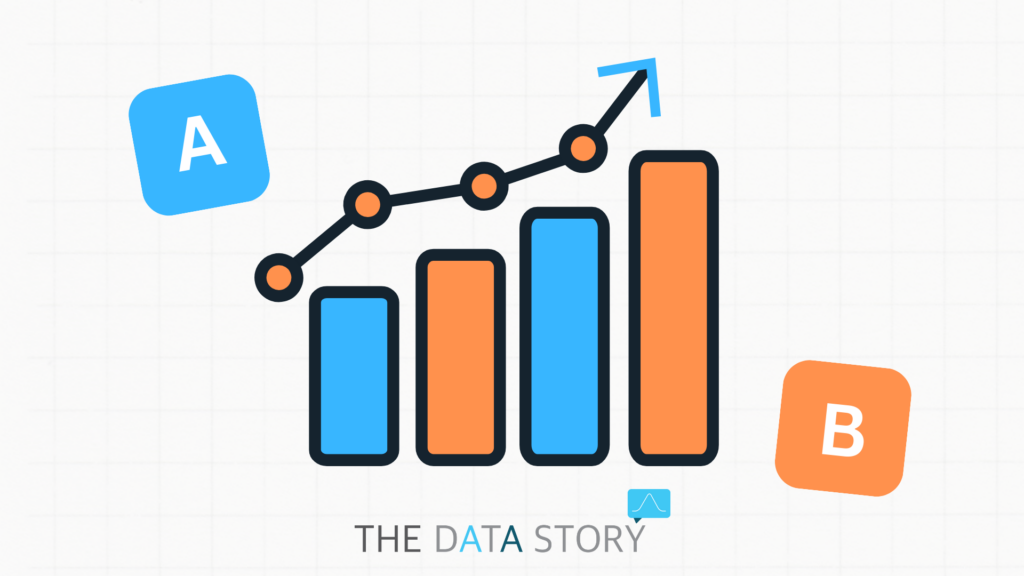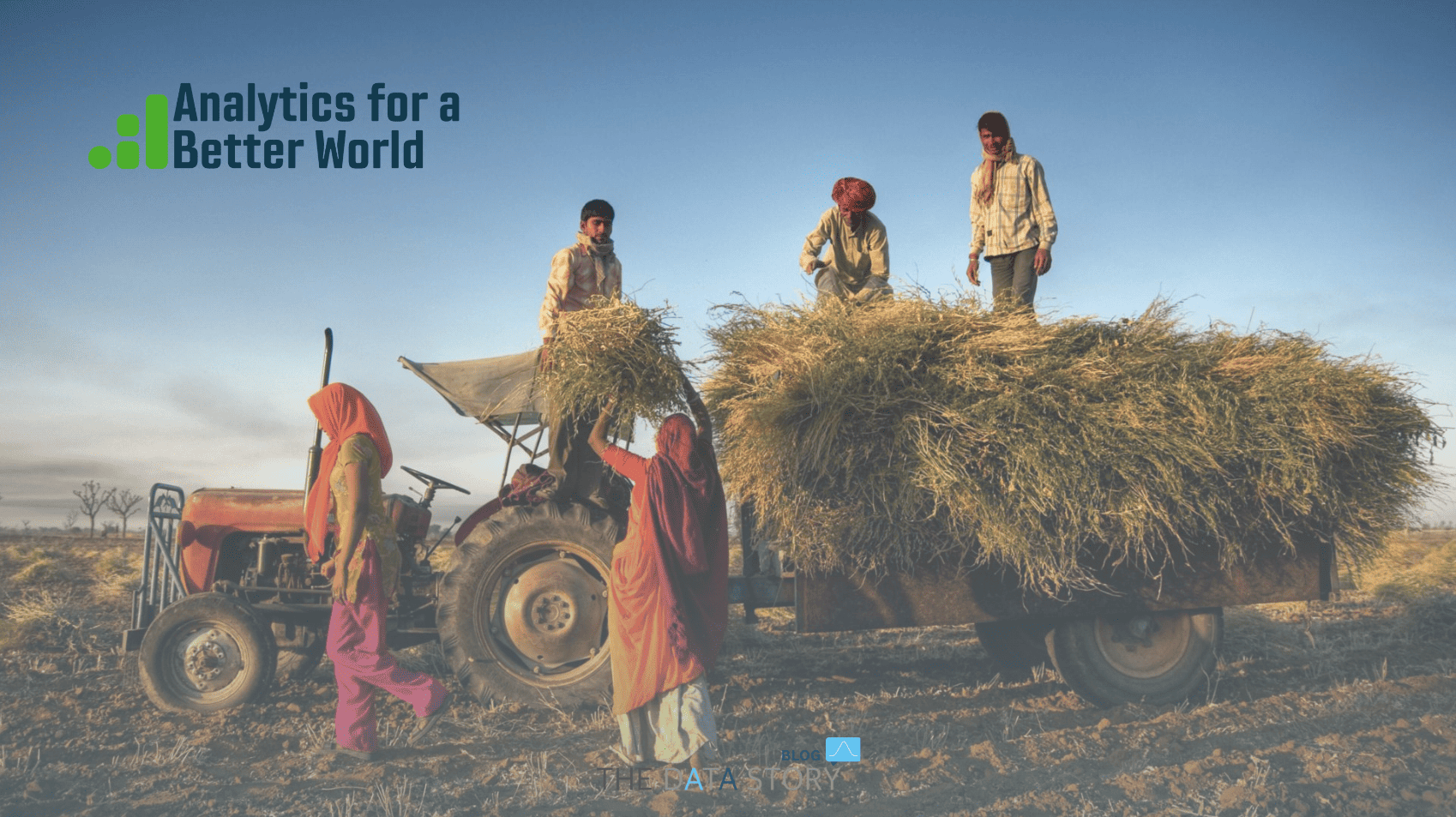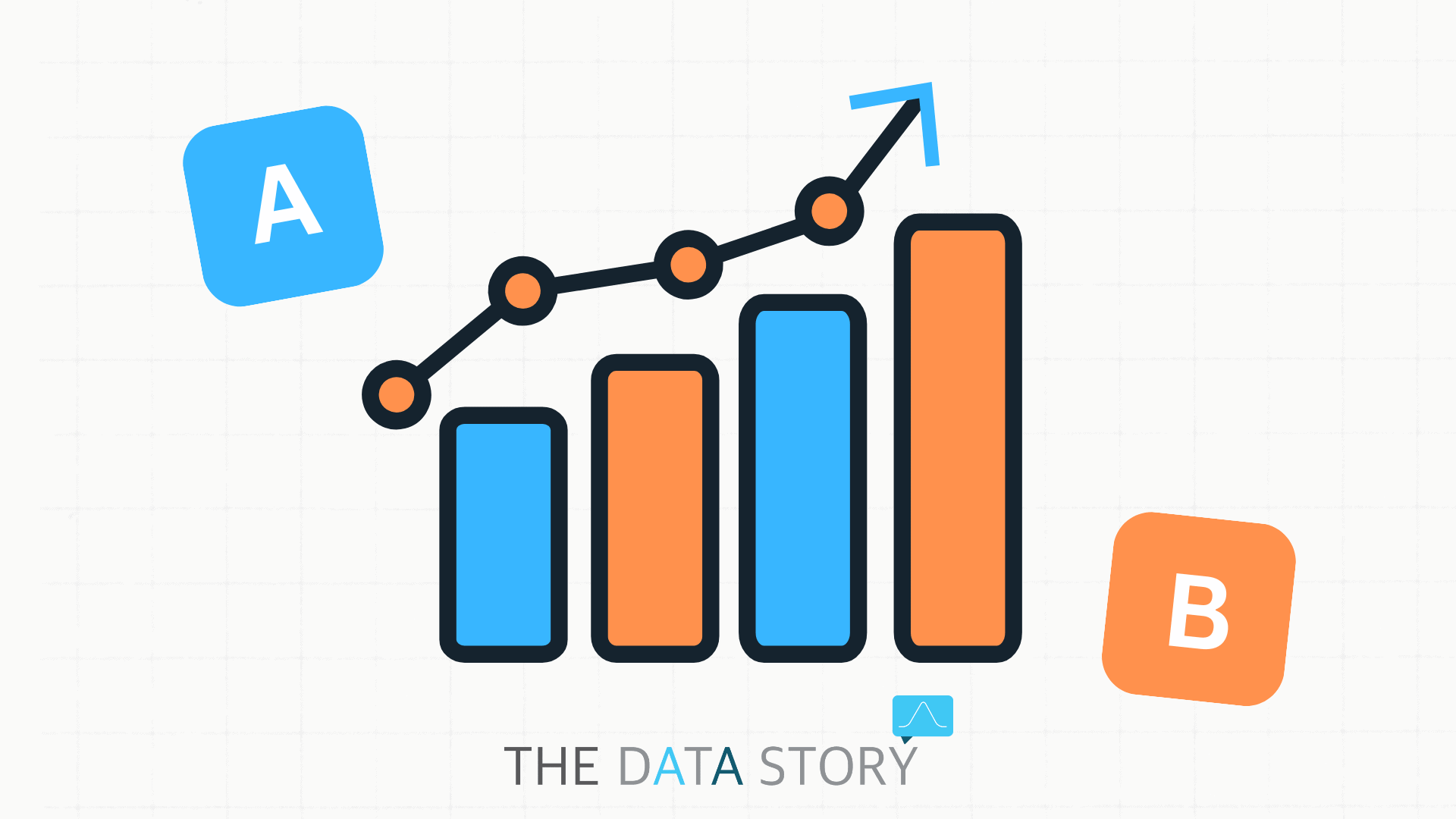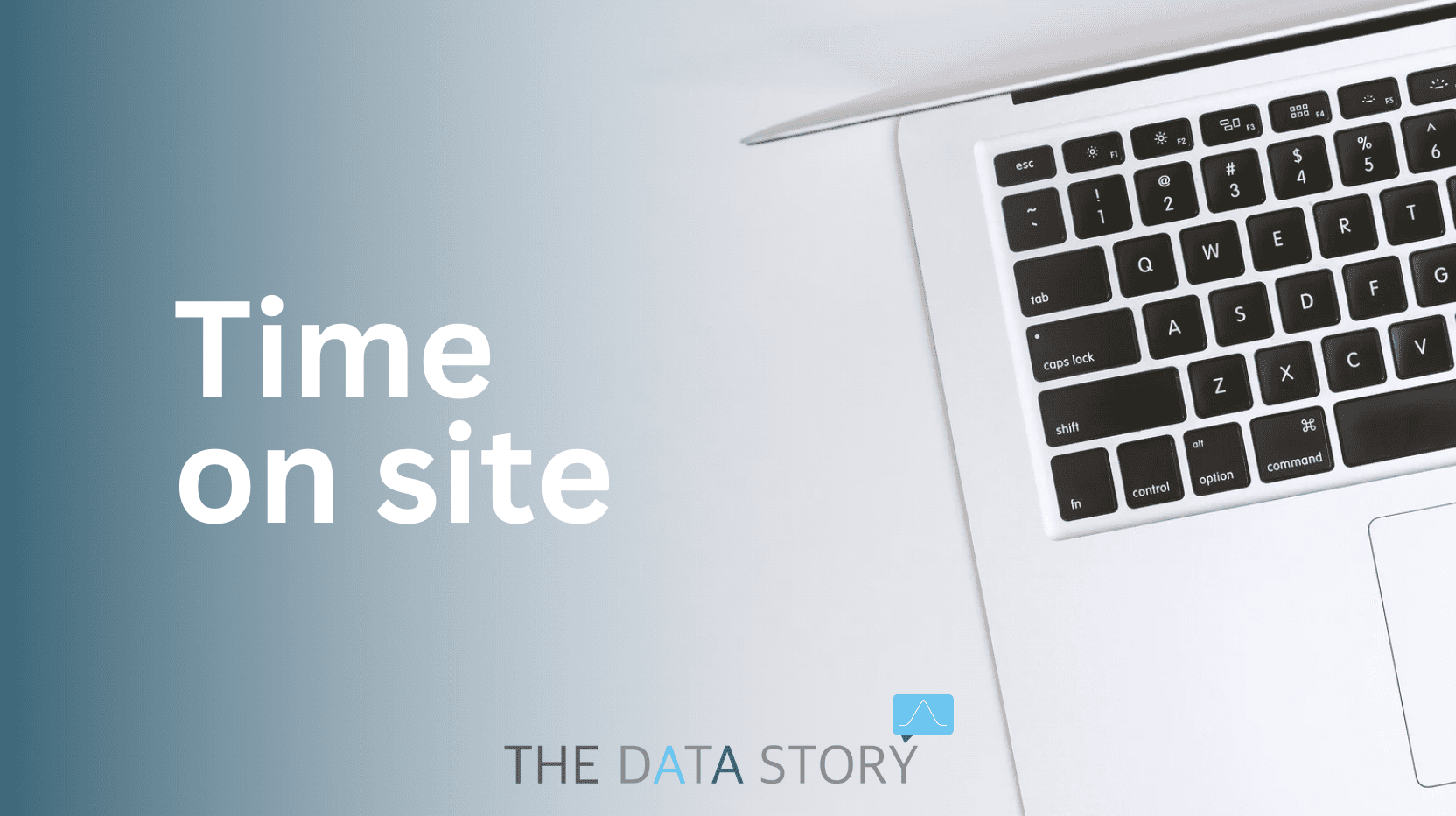# A/B testing: In statistics we trust

Although the simple mention of the word ‘statistics’ might make your skin crawl, statistics are an essential tool of A/B testing. We created a simple cheat sheet to help you navigate the basic terminology of statistics with A/B testing.

## 1 – Populations and samples

In statistics, we want to find results that apply to an entire population of people or things. However, in most cases, it is impossible to collect data from the entire population. This is why we collect data from a small subset of the population, called a sample.

## 2 – Mean

The mean is the simple mathematical average of a set of numbers. If you, for example, sell lamps and your customers’ purchases are as follows:

• Customer A: purchased 2 lamps
• Customer B: 2 lamps
• Customer C: 3 lamps
• Customer D: 2 lamps

You would calculate the mean by adding the values (lamps purchased) and dividing that sum by the number of values measured (number of customers): (2+2+3+2)/4 = 2.25.

## 3 – Standard deviation vs. standard error

These two metrics are very often mixed up. In short, standard deviation is about your data and standard error is about your sample.

The standard deviation is a measure of how well your mean represents your data. It indicates how much members differ from the mean value of the group. Conversely, the standard error tells you how well your sample represents the total population.

## 4 – Confidence intervals

Confidence intervals are boundaries within which we believe the true value of the mean will fall. The purpose of confidence intervals is to establish a range within which values of a population will fall with certain probability. Usually, we look at 95% confidence intervals and sometimes 99%. This means that we expect 95% or 99% of values to fall within the range of the confidence interval.

## 5 – Null Hypothesis vs. experimental hypothesis

An experimental hypothesis is the prediction that your experimental manipulation will have some effect or that certain variables will relate to each other. In contrast, the null hypothesis is the prediction that you are wrong and that the predicted effect doesn’t exist. In other words, the experimental hypothesis is something a researcher tries to prove whereas the null hypothesis is something the researcher tries to disprove.

## 6 – Type I and Type II errors

A type 1 error, also called a false-positive, occurs when we believe that there is an effect in our population, when in fact there isn’t. The opposite is a Type II error, a false-negative, which occurs when we believe that there is no effect on the population when in reality there is.## 7 – p-value

The p-value is the probability of observing an outcome equally or more extreme than the one observed in the test, assuming that the null hypothesis is true. The smaller the p-value, the more certain we are that we should reject the null hypothesis.

## 8 – Statistical Significance

The result is statistically significant when the p-value is smaller than the significance level. The significance level (𝛂) is the probability of rejecting the null hypothesis when it is actually true. In other words, it is the probability of wrongly rejecting the null hypothesis. For example, a significance level of 0.05 indicates a 5% risk of making the wrong assumption: rejecting the null hypothesis when, in reality, it is true.

## 9 – Statistical Power

Statistical power is the probability of observing a statistically significant effect if there is indeed one. In other words, it allows you to detect a difference between test variations. That is, if that difference actually exists.

We hope this A/B testing blog will help you along! Part 1 of our A/b testing blog can be found here.

Feel like reading more data stories? Then take a look at our blog page. Always want to stay up to date? Be sure to follow us on LinkedIn!

## Need some help?### Sophie Caro

“Data is een van de meest waardevolle bezittingen die je kunt hebben. Ik draag graag bij aan de groei en ontwikkeling van bedrijven door hen te helpen hun gegevens om te zetten in inzichten.”## More Data storiesData stories

## Analytics for a Better World

I, Sophie, attended the Analytics for a Better World (ABW) annual conference at Amsterdam Business School on May 24th. This event brought together speakers and panelists from different groups: nonprofits,...Data stories

## GA4 Data Alerts in Slack

In this blogpost you will read about a Data Alerts system in Slack: a Slackbot that alerts users when it finds inconsistencies in data, using predefined BigQuery queries. After a...Data stories

## A/B testing: In statistics we trust

Although the simple mention of the word ‘statistics’ might make your skin crawl, statistics are an essential tool of A/B testing. We created a simple cheat sheet to help you...Data stories

## Don't focus on time-on-site

At The Data Story we try to make data understandable. To us, that is one of the most important aspects of turning data into gold. Once you understand what the...Data stories

## Product experimenting: which test should you choose?

Navigating the world of Product experimenting can sometimes be challenging. Which test should you choose? How many users should you have in it? How do you make sure your results...Data stories